### Home > CALC > Chapter 5 > Lesson 5.2.4 > Problem5-97

5-97.
1. Sketch each of the following piecewise functions. Then, determine if the functions are continuous and differentiable over all reals. Homework Help ✎

1.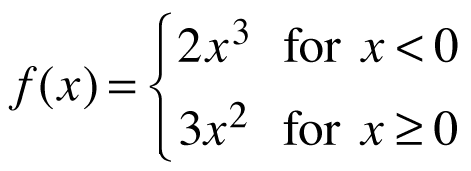2.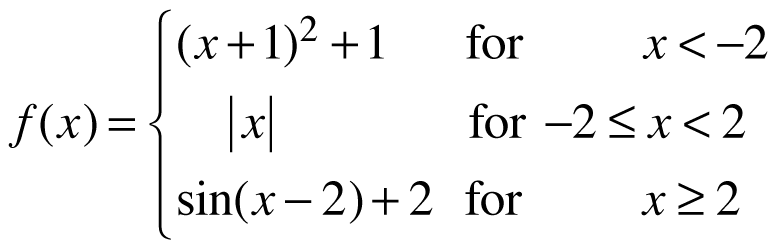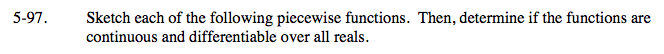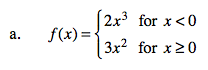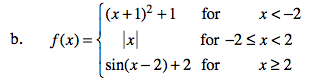To test if the function is continuous at the boundary point, use the three conditions of continuity:

2. f(a) exists.

To test if the function is differential at the boundary point, use the same three conditions on the derivative.

2. f '(a) exists. (Note, differentiability implies continuity.)

$3. \ f'(a)=\lim_{x\rightarrow a}f'(x)$

Notice that part (b) has two boundary points, so you will have to run these tests twice.